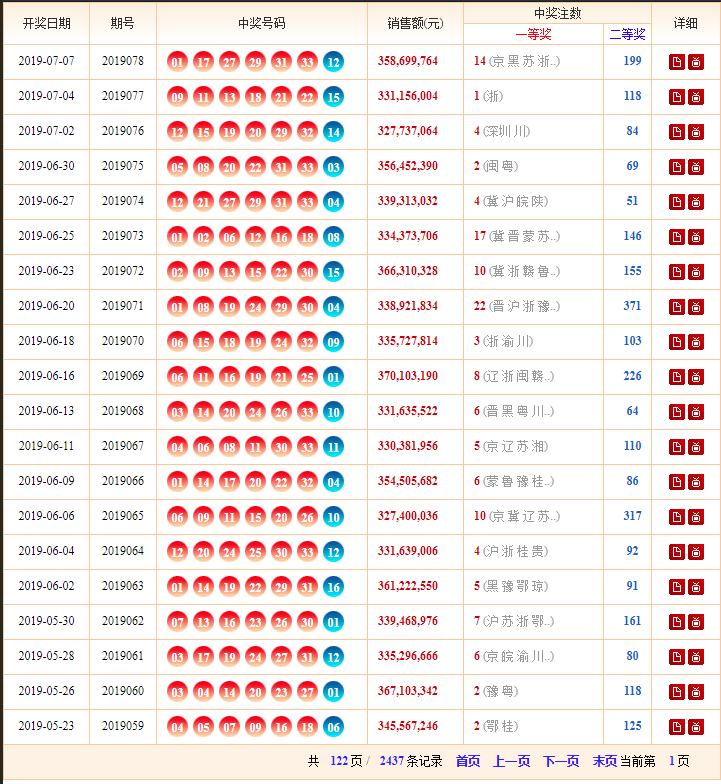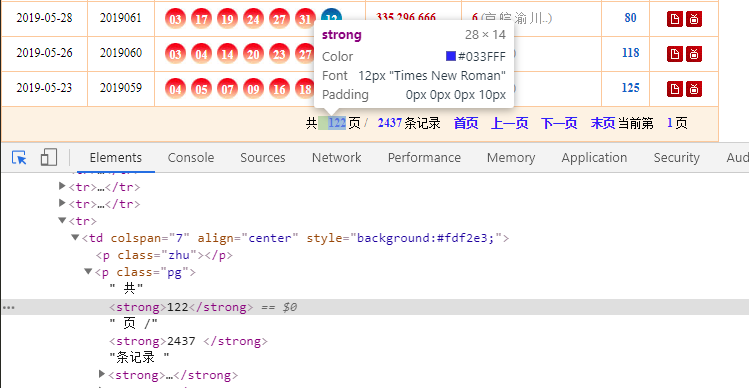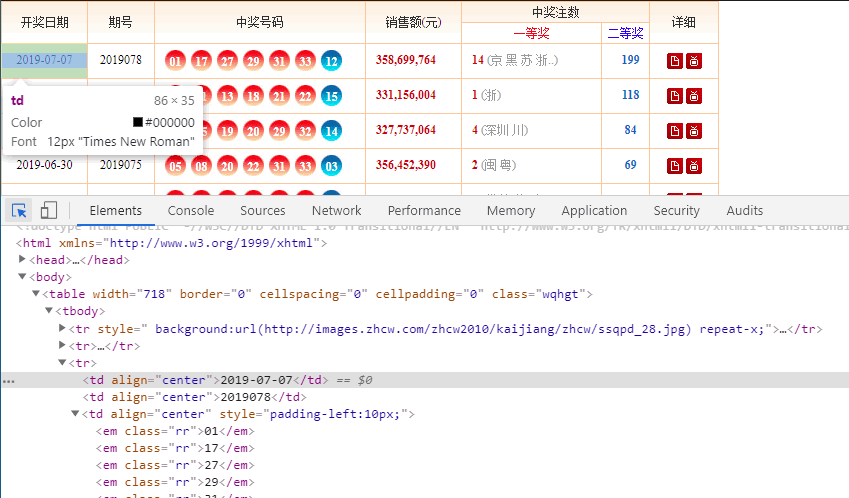分析了16年的福利彩票记录，原来可以用Python这么买彩票0 引言
1 环境
2 需求分析
3 代码实现
4 后记

Python版本：3.7.3

2 需求分析

import random

def ball():
ball_list=[]
while 1:
a=random.randint(1,33)
if a not in ball_list:
ball_list.append(a)
if len(ball_list)==6:
break

ball_list.sort()
ball_list.append(random.randint(1,16))
print(ball_list)

ball()

http://kaijiang.zhcw.com/zhcw/html/ssq/list_1.htmlhttp://kaijiang.zhcw.com/zhcw/html/ssq/list_1.html

http://kaijiang.zhcw.com/zhcw/html/ssq/list_2.html

http://kaijiang.zhcw.com/zhcw/html/ssq/list_3.html

http://kaijiang.zhcw.com/zhcw/html/ssq/list_4.html

http://kaijiang.zhcw.com/zhcw/html/ssq/list_5.html

url_part = 'http://kaijiang.zhcw.com/zhcw/html/ssq/list'
url = url_part + '_' + str(i) + '.html'